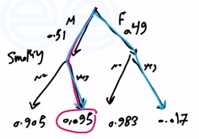Need Help?

Subscribe to Probability

###### \${selected_topic_name}
• Notes
• Comments & Questions

In Orange County, $51\%$ of the adults are males. (It doesn't take too much advanced
mathematics to deduce that the other $49\%$ are females.) One adult is randomly selected
for a survey involving credit card usage.

a. Find the prior probability that the selected person is a male.

b. It is later learned that the selected survey subject was smoking a cigar. Also, $9.5\%$
of males smoke cigars, whereas $1.7\%$ of females smoke cigars (based on data
from the Substance Abuse and Mental Health Services Administration). Use this
additional information to find the probability that the selected subject is a male.

males $\rightarrow 51\%$
females $\rightarrow 49\%$

(a) $P(M)=51 \%$(b) $P(M|S)=\frac{P(S | M) P(M)}{P(S)}$

$=\frac{0,095 \times 0.51}{P(S | M) P(M)+P(S | F) P(F)}=0.85$

No comments yet